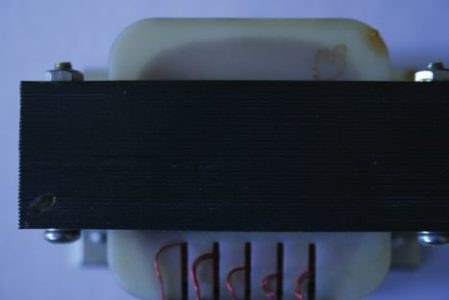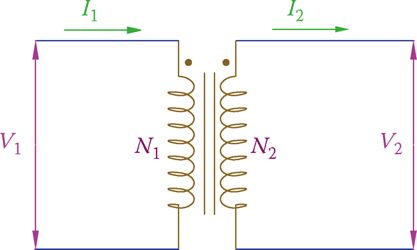# Ideal Transformer | Theory | Equations | Example Problems

Want create site? Find Free WordPress Themes and plugins.

In all transformers, single phase or three phase, the primary winding gets energy from the mains and the secondary winding is connected to the load(s). If the secondary circuit is turned off by a switch, there is no load on the secondary winding.

## Ideal Transformer Theory

Nevertheless, the primary winding is still connected to electricity and forms a closed circuit. In this case, the primary winding behaves as a coil with a core; a current flows through it that (1) warms the winding and (2) warms the core as a result of eddy currents and hysteresis.

Eddy current is a local movement of electrons in a ferromagnetic material when placed in a magnetic field.

In a solid piece of iron or other ferromagnetic metal, there can be many local circuits of eddy current in various directions, depending on the variation of the magnetic field. This unwanted current not only warm up a core, it consumes energy and also affects the magnetic property of the core metal.

To reduce the eddy currents and its effects, all the core materials of transformers and motors are made up of laminated metal.

Each lamination has a layer of paint or nonconductive wax on it so that it is electrically isolated from its neighbors. In this way, eddy currents are limited to those only inside of one laminated metal.

Figure 1 shows what a laminated metal looks like. This greatly reduces the amount of energy loss by eddy current and the resulting heat.Figure 1 Laminated metal for transformers and motors.

Nevertheless, eddy currents cannot be entirely stopped and the associated energy loss cannot be reduced to zero.

There are other types of loss in transformers, such as hysteresis and mechanical loss (energy changed to noise). But these are relatively smaller than eddy current loss in a well-designed transformer.

The loss due to the current in the winding(s) is called copper loss, and the losses due to eddy currents, hysteresis, and so on are referred to as core loss because they occur in the transformer core, and they are independent of how much the current is (but they increase as frequency goes up).

Copper loss: Amount of power loss in a transformer that corresponds to the resistance of the wire winding and it depends on the load current (the percentage of loading).

Core loss: Amount of power loss in a transformer that corresponds to the quality of design and core material and is independent of the load current.

## Ideal Transformer Example

To develop the electrical relationships between the primary and secondary transformers, all losses are assumed to be zero. This simplifies the relationships and facilitates developing the pertinent equations. Such an assumed transformer in which all the losses are neglected is called an ideal transformer.

Ideal transformer: When in a transformer all the losses are assumed to be zero and, as a result, input power equals output power.

In an ideal transformer, the output power and the input power are equal. That is, all power received by the primary winding is delivered to the secondary winding.

In dealing with any device, including transformers, one needs to bear in mind that it is always the load that determines how much power is required.

In an ideal transformer, all the losses are assumed to be zero. As a result, the power in the primary side is equal to the secondary side power.

## Voltage Relationship in Ideal Transformer

Referring to Figure 2, the primary winding is connected to a supply voltage V1 and the secondary voltage V2 is applied to a load.

In general, a load can be resistive, inductive, capacitive, or a combination of these. A current I2 will flow in the secondary winding. On the basis of this current, the primary winding carries a current I1.Figure 2 Number of turns N, voltage V and current I in the primary and secondary circuits of a transformer.

The relationship between the primary and secondary voltages is based on the ratio of the number of turns in the primary and secondary windings.

The turns ratio in a transformer is the ratio of turns in the primary winding to that of the secondary winding and is denoted by a.

Turns ratio: Ratio of the number of turns in the secondary and primary windings of a transformer.

The following equation is the fundamental relationship for an ideal transformer:

$\begin{matrix} \frac{{{V}_{1}}}{{{V}_{2}}}=\frac{{{N}_{1}}}{{{N}_{2}}}=a & {} & \left( 1 \right) \\\end{matrix}$

Where V1 and V2 are the primary and secondary voltages, respectively; N1 and N2 are the number of turns in the primary and secondary windings, and a is the turns ratio.

For most transformers, the turns ratio is fixed and cannot change.

When a > 1, the secondary voltage is smaller than the primary voltage, thus a step-down transformer.

When a < 1, the secondary voltage is larger than the primary voltage and the transformer is a step-up transformer.

Equation 1 shows that a transformer with a given turns ratio, for instance, 10, can divide its input voltage by 10 at the secondary winding. This transformer can theoretically also multiply its input voltage by 10.

For instance, if the side with the lower number of turns is connected to 120 V, the secondary winding has 1200 V at its terminals. This implies an important and serious issue: in working with a transformer, special care must be taken for its correct connection to the source and loads. The wrong connection can easily lead to damage and injuries.

In a step-up transformer, the secondary voltage is higher than the primary voltage. In a step-down transformer, it is the reverse.

Take note that although theoretically, one can connect a transformer for stepping up or stepping down a given voltage, and there are transformers designed for working both ways, in practice, design considerations such as wire thickness and transformer power rating determine the limitation for the use of a transformer.

A transformer cannot necessarily be connected to any arbitrary voltage or in an arbitrary fashion. In working with a transformer, special care must be taken for correct connection of its primary and secondary to the outside circuits.

Ideal Transformer Example Problem 1

The primary winding of a transformer has 1000 turns. If this transformer is to be used for changing 120 V input to 24 V output, how many turns are needed for the secondary winding?

Solution

It follows from Equation 1 that the number of secondary winding turns is

${{N}_{2}}=\frac{{{V}_{2}}}{{{V}_{1}}}\times {{N}_{1}}=\frac{24}{120}\times 1000=200$

In using transformers, often the turns ratio is mentioned in the form of a:1 (a to 1) or 1:a (1 to a). For example, in a 2:1 transformer the primary voltage is twice the secondary voltage.

In conjunction with Equation 1, one can understand that in a transformer the ratio

$\frac{V}{N}=\frac{{{V}_{1}}}{{{N}_{1}}}=\frac{{{V}_{2}}}{{{N}_{2}}}$

is a constant. This constant is called volts per turn and determines how many volts there are per each turn of either the primary or the secondary winding. The use of this constant is in the design stage of a transformer.

Volts per turn: Number indicating the value of volts for each turn of winding in a transformer. This value can be obtained from either the primary side values or the secondary values by dividing the voltage by the number of turns.

## Current Relationship in Ideal Transformer

The relationship between the primary and secondary currents for an ideal transformer is based on the power relationship; that is, the power in the primary side is equal to the power consumed in the secondary side.

Always, in AC electricity the consumed power refers to the apparent power, the product of voltage and current. Writing

\begin{align} & S={{V}_{1}}{{I}_{1}}={{V}_{2}}{{I}_{2}} \\ & \\ & Leads\text{ }to \\ & \\ & \begin{matrix} \frac{{{V}_{1}}}{{{V}_{2}}}=\frac{{{I}_{2}}}{{{I}_{1}}} & {} & {} \\ or & {} & \left( 2 \right) \\ \frac{{{I}_{2}}}{{{I}_{1}}}=\frac{{{N}_{1}}}{{{N}_{2}}}=a & {} & {} \\\end{matrix} \\\end{align}

This equation also implies that in a transformer the side with the higher voltage has a smaller current and vice versa.

The secondary current I2 can always be found from the secondary voltage and the load impedance connected to the secondary. Having the current in the secondary, then the current I1 in the primary can be found. This is for an ideal transformer, and I1 represents the current in the primary due to the load only.

If there is no load connected to the secondary side, this current is zero, too. In other words, in an ideal transformer, the current I1 as obtained from Equation 2 does not include the no-load current and the core loss currents (due to eddy currents in the core, mentioned earlier) that exist in a real transformer.

Because the current in the secondary and primary windings are not the same, the wire sizes for the two windings are not the same.

Very Important

The current in the winding with the lower number of turns is always higher than the current in the other winding. Thus, the lower voltage (higher current) side always has a thicker wire than the higher voltage (lower current) side. This can be a good way to judge the connections if in doubt.

For a step-down transformer, the secondary current is higher and the winding wire is thicker than that of the primary winding.

Ideal Transformer Example Problem 2

A step-down transformer is used to change 220 to 110 V. If a resistive load consuming 500 W is connected to the 110 V side, what is the current due to this load in the secondary and primary windings?

Solution

Because this is a 2:1 transformer, current in the primary winding is half of the current in the secondary winding. From the power of the load and the voltage in the secondary side, the current I2 can be found

${{I}_{2}}=\frac{P}{{{V}_{2}}}=\frac{500}{110}=4.54A$

From which the current in the primary side follows

${{I}_{1}}=0.5\times 4.54=2.27A$

Did you find apk for android? You can find new Free Android Games and apps.